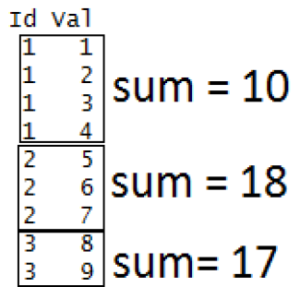# Apply a Function over a Ragged Array in R Programming – tapply() Function

`tapply()` function in R Language is used to apply a function over a subset of vectors given by a combination of factors

Syntax: tapply(vector, factor, fun)

Parameters:
vector: Created Vector
factor: Created Factor
fun: Function to be applied

Example 1:

 `# R Program to apply a function ` `# over a data object ` ` `  `# Creating Factor ` `fac <``-` `c(``1``, ``1``, ``1``, ``1``, ``2``, ``2``, ``2``, ``3``, ``3``) ` ` `  `# Created Vector ` `vec <``-` `c(``1``, ``2``, ``3``, ``4``, ``5``, ``6``, ``7``, ``8``, ``9``)  ` ` `  `# Calling tapply() Function ` `tapply(vec, fac, ``sum``) `

Output:

``` 1  2  3
10 18 17
```

This is how above code works:Example 2:

 `# R Program to apply a function ` `# over a data object ` ` `  `# Creating Factor ` `fac <``-` `c(``1``, ``1``, ``1``, ``1``, ``2``, ``2``, ``2``, ``3``, ``3``) ` ` `  `# Created Vector ` `vec <``-` `c(``1``, ``2``, ``3``, ``4``, ``5``, ``6``, ``7``, ``8``, ``9``)  ` ` `  `# Calling tapply() Function ` `tapply(vec, fac, prod) `

Output:

``` 1   2   3
24 210  72
```

My Personal Notes arrow_drop_upCheck out this Author's contributed articles.

If you like GeeksforGeeks and would like to contribute, you can also write an article using contribute.geeksforgeeks.org or mail your article to contribute@geeksforgeeks.org. See your article appearing on the GeeksforGeeks main page and help other Geeks.

Please Improve this article if you find anything incorrect by clicking on the "Improve Article" button below.

Article Tags :

1

Please write to us at contribute@geeksforgeeks.org to report any issue with the above content.